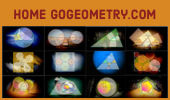# Geometry from the Land of the Incas is a free educational website, aimed mainly at high school and college students with the goal of raising interest in Euclidean geometry. Antonio Gutierrez.Geometry Problem 1492.
Right Triangle, Altitude, Incenters, Angle, Measurement.

Geometry Problem 1491.
Cyclic Quadrilateral, Diagonal, Incircle, Angle, Measurement.

Geometry Problem 1490.
Triangle, Cevian, Incircle, Tangent, Measurement.

Geometry Problem 1489.
Right Triangle, Angle Bisectors, Perpendicular, Measurement.

Geometry Problem 1488.
Right Triangle, Altitude, Incircle, Tangent, Measurement.

Geometry Problem 1487.
Right Triangle, Altitude, Incircle, Tangent, Measurement.

Geometry Problem 1486.
Right Triangle, Altitude, Incircle, Tangent, Measurement.

Geometry Problem 1485.
Triangle, Orthocenter, Altitude, Circle, Diameter, Tangent, Measurement.

Geometry Problem 1484.
Right Triangle, Altitude, Incircles, Inradius, Measurement.

Geometry Problem 1483.
Isosceles Right Triangle, Excenter, Perpendicular, Measurement.

Geometry Problem 1482.
Right Triangle, Perpendicular, Double Angle, Measurement.

Dynamic Geometry 1481.
Five Tangential or Circumscribed Quadrilaterals, Pitot Theorem, Congruence, Step-by-step Illustration. GeoGebra, iPad.

Geometry Problems
Over 1400 Visually Stimulating Problems. College, High School

Inca Geometry.
Machu Picchu, Cuzco, Nazca Lines, and more.

Word Cloud or Tag Cloud Index
Weighted list in visual design

Geometria en Espanol, Teoremas y Problemas en Espanol.
(Spanish-language version)

Inca Gold, Mining in the World.
Index.

Go Geometry from the Land of the Incas- Table of Content

 Home | Translate | Email | by Antonio Gutierrez Last updated: Dec 30, 2020